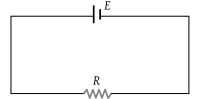NEET Physics Current Electricity Questions Solved

Consider the circuit given here with the following parameters E.M.F. of the cell = 12 V. Internal resistance of the cell = 2 Ω. Resistance R = 4 Ω Which one of the following statements in true(1) Rate of energy loss in the source is = 8 W

(2) Rate of energy conversion in the source is 16 W

(3) Power output in is = 8 W

(4) Potential drop across R is = 16 V

Explanation is a part of a Paid Course. To view Explanation Please buy the course.

Difficulty Level: